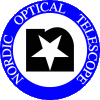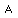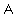# ALFOSC Arc Lamps used with CCD7 and CCD8

## Mounted lamps

For more than a decade we have the following lamps installed in ALFOSC: Halogen, Helium, Neon, Thorium-Argon (faint). See this page for typical exposure times for the different grisms. Note that Helium and Neon can be switched-on at the same time, as in fact any other combination of installed lamps.

## Lamps and arc maps

Spectral lamps from Osram: Ne, He, Cs, Zn
Hollow cathode lamps from Cathodeon/Heraeus: Nb, Cu, Th-Ar, Fe-Ar

The Osram Cs and Zn lamps use Ar as a filler gas. The Cathodeon Nb and Th lamps also have Ar as a filler gas, while the Cu lamp has Ne as a filler. All this means that the Cs, Zn, Nb and Th lamps all give Ar lines as well as lines from the appropriate element while the Cu lamp gives Ne lines as well as Cu lines.

Beware! the lines from Nb and Cu are all faint and generally require a integration of up to 20 minutes to detect them!

Wavelength calibration.
NB: These arc maps give no indication of exposure times needed!!

Grism #3
3200-6700
plot 1
plot 2
plot 1
plot 2
plot

Grism #4
3200-9100
plot 1
plot 2
plot 1
plot 2
plot 1
plot 2
plot
note

plot
note

Grism #5
5000-10250
plot plot 1
plot 2
plot 1
plot 2

Grism #6
3200-5500
plot not
useful
plot plot
note

plot
note

Grism #7
3850-6850
plot 1
plot 2
plot 1
plot 2
plot plot
note

plot
note

Grism #8
5825-8350
plot plot 1
plot 2
plot 1
plot 2
plot
note

plot
note

Grism #9
3300-10350
+ CD #10

plot

Grism #9
3900-10350
+ CD #11

plot

Grism #9
5200-11000
+ CD #12

plot

Grism #10
3300-10550
plot 1
plot 2
plot 1
plot 2
plot 1
plot 2
plot1 plot2
note

plot1 plot2
note

Grism #11
3900-9950
plot 1
plot 2
plot 1
plot 2
plot 1
plot 2

Grism #12
5100-11000
plot plot plot

Grism #13
4800-5800
+ order sorter
plot plot plot

Grism #13
3350-8650
+ CD #10

plot

Grism #14
3275-6125
plot 1
plot 2
not
useful
plot

Grism #15
3200-9125
plot 1
plot 2
plot 1
plot 2
plot 1
plot 2

Grism #16
3500-5060

plot

Grism #17
6350-6850

plot
plot 1
plot 2

Grism #18
3520-5190
plot
plot

Grism #19
4510-6770
plot plot plot

Grism #20
5850-9840
plot plot plot

Also: He,Ne,Ar Line List (42k gzipped postscript), and an overview of IRAF line lists.

Documentation on See here how to change the labels after having changed the lamps.

 Back to top Last modified: 15-Apr-2016